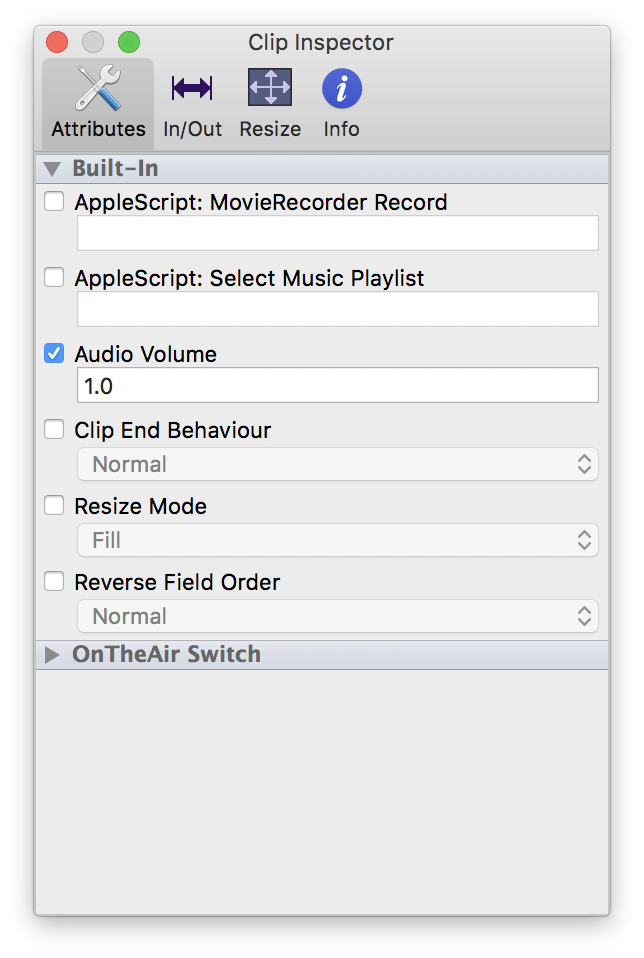# Clip Audio Volume: possible values in OnTheAir Node

The Audio Volume can be set for each clip In OnTheAir Node. You can change the audio volume using the Clip Inspecto when you select one (or more) clip(s) in OnTheAir Live or OnTheAir Manager.Since it is a standard attribute, we can not limit its values.  Even though you can basically enter anything in there, only some values will be accepted by OnTheAir Node.

You can set any value between 0.0 (-99dB) and 2.0 (+6dB).

Note that it is not linear. The formula used is to transform into dB is: log10 (AttributeValue) * 20

So here are a few values:

• 0.0 = -99.0dB
• 0.1 = -20.0dB
• 0.2 = -14.0dB
• 0.3 = -10.5dB
• 0.4 = -8.0dB
• 0.5 = -6.0dB
• 0.6 = -4.4dB
• 0.7 = -3.0dB
• 0.8 = -1.9dB
• 0.9 = -0.9dB
• 1.0 = 0.0dB
• 1.1 = +0.8dB
• 1.2 = +1.6dB
• 1.3 = +2.3dB
• 1.4 = +2.9dB
• 1.5 = +3.5dB
• 1.6 = +4.0dB
• 1.7 = +4.6dB
• 1.8 = +5.1dB
• 1.9 = +5.5dB
• 2.0 = +6.0dB